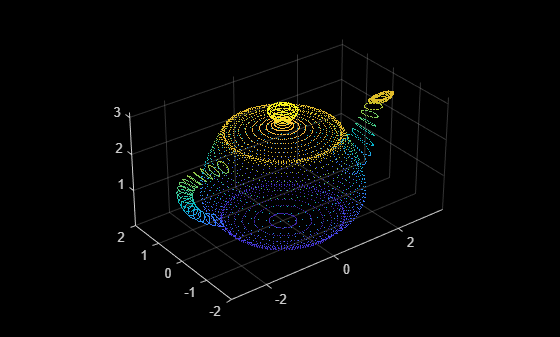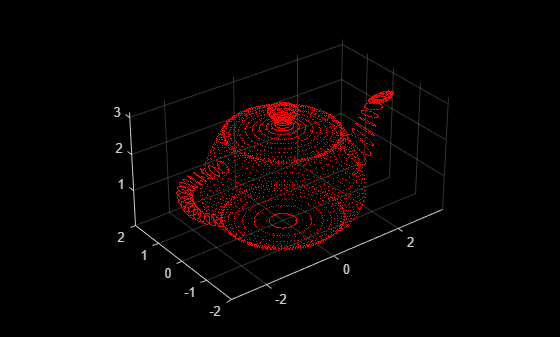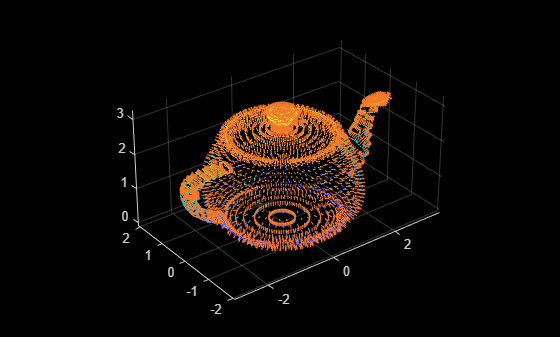Documentation

pointCloud

Object for storing 3-D point cloud

Description

The pointCloud object creates point cloud data from a set of points in 3-D coordinate system. The point cloud data is stored as an object with the properties listed in Properties. Use Object Functions to retrieve, select, and remove desired points from the point cloud data.

Creation

Description

ptCloud = pointCloud(xyzPoints) returns a point cloud object with coordinates specified by xyzPoints.

example

ptCloud = pointCloud(xyzPoints,Name,Value) creates a pointCloud object with properties specified as one or more Name,Value pair arguments. For example, pointCloud(xyzPoints,'Color',[0 0 0]) sets the Color property of the point xyzPoints as [0 0 0]. Enclose each property name in quotes. Any unspecified properties have default values.

Input Arguments

expand all

3-D coordinate points, specified as a M-by-3 or M-by-N-by-3 array. The 3-D coordinate points specify the x, y, and z positions of a point in the 3-D coordinate space. This argument sets the Location property.

Data Types: single | double

Output Arguments

expand all

Point cloud, returned as a pointCloud object with the properties listed in Properties.

Properties

expand all

This property is read-only.

Position of the points in 3-D coordinate space, specified as an M-by-3 or M-by-N-by-3 array. Each entry specifies the x, y, and z coordinates of a point in the 3-D coordinate space. You cannot set this property as a name-value pair. Use the xyzPoints input argument.

Data Types: single

Point cloud color, specified as an M-by-3 or M-by-N-by-3 array. Use this property to set the color of points in point cloud. Each entry specifies the RGB color of a point in the point cloud data. Therefore, you can specify the same color for all points or a different color for each point.

• The specified RGB values must lie within the range [0, 1], when you specify the data type for Color as single or double.

• The specified RGB values must lie within the range [0, 255], when you specify the data type for Color as uint8.

 Coordinates Valid assignment of Color M-by-3 array M-by-3 array containing RGB values for each pointM-by-N-by-3 array M-by-N-by-3 array containing RGB values for each pointData Types: uint8

Surface normals, specified as a M-by-3 or M-by-N-by-3 array. Use this property to specify the normal vector with respect to each point in the point cloud. Each entry in the surface normals specifies the x, y, and z component of a normal vector.

CoordinatesSurface Normals
M-by-3 arrayM-by-3 array, where each row contains a corresponding normal vector.
M-by-N-by-3 arrayM-by-N-by-3 array containing a 1-by-1-by-3 normal vector for each point.

Data Types: single | double

Grayscale intensities at each point, specified as a M-by-1 vector or M-by-N matrix. The function maps each intensity value to a color value in the current colormap.

CoordinatesIntensity
M-by-3 arrayM-by-1 vector, where each row contains a corresponding intensity value.
M-by-N-by-3 arrayM-by-N matrix containing intensity value for each point.

Data Types: single | double | uint8

This property is read-only.

Number of points in the point cloud, stored as a positive integer.

This property is read-only.

Range of coordinates along x-axis, stored as a 1-by-2 vector.

This property is read-only.

Range of coordinates along y-axis, stored as a 1-by-2 vector.

This property is read-only.

Range of coordinates along z-axis, stored as a 1-by-2 vector.

Object Functions

 findNearestNeighbors Find nearest neighbors of a point in point cloud findNeighborsInRadius Find neighbors within a radius of a point in the point cloud findPointsInROI Find points within a region of interest in the point cloud removeInvalidPoints Remove invalid points from point cloud select Select points in point cloud

Examples

collapse all

Read the 3-D coordinate points into the workspace.

Create a point cloud object from the input point coordinates.

ptCloud = pointCloud(xyzPoints);

Inspect the properties of the point cloud object.

ptCloud
ptCloud =
pointCloud with properties:

Location: [5184×3 single]
Color: []
Normal: []
Intensity: []
Count: 5184
XLimits: [-3 3.4338]
YLimits: [-2 2]
ZLimits: [0.0016 3.1437]

Display the point cloud by using pcshow.

pcshow(ptCloud)Modify Color of Point Cloud Data

Create an RGB color array of size same as the size of the point cloud data. Set the point colors to Red.

cmatrix = ones(size(ptCloud.Location)).*[1 0 0];

Create the point cloud object with the color property set to the RGB color array.

ptCloud = pointCloud(xyzPoints,'Color',cmatrix);
pcshow(ptCloud)Add Surface Normals to Point Cloud Data

Compute surface normals corresponding to the point cloud data using pcnormals.

normals = pcnormals(ptCloud);

Create point cloud object from input point coordinates. Add the computed surface normals to point cloud object.

ptCloud = pointCloud(xyzPoints,'Normal',normals);

Display the point cloud and plot the surface normals.

pcshow(ptCloud)
x = ptCloud.Location(:,1);
y = ptCloud.Location(:,2);
z = ptCloud.Location(:,3);
u = normals(:,1);
v = normals(:,2);
w = normals(:,3);
hold on
quiver3(x,y,z,u,v,w);
hold offTips

The pointCloud object is a handle object. If you want to create a separate copy of a point cloud, you can use the MATLAB® copy method.

 ptCloudB = copy(ptCloudA)

If you want to preserve a single copy of a point cloud, which can be modified by point cloud functions, use the same point cloud variable name for the input and output.

 ptCloud = pcFunction(ptCloud)